MathScore EduFighter is one of the best math games on the Internet today. You can start playing for free!

## Circle Circumference - Sample Math Practice Problems

The math problems below can be generated by MathScore.com, a math practice program for schools and individual families. References to complexity and mode refer to the overall difficulty of the problems as they appear in the main program. In the main program, all problems are automatically graded and the difficulty adapts dynamically based on performance. Answers to these sample questions appear at the bottom of the page. This page does not grade your responses.

See some of our other supported math practice problems.

### Complexity=4, Mode=exact

Find the circumference in terms of π and the unit of measurement. Type "pi" in for π so that "7π m" would look like "7pi m".

 1.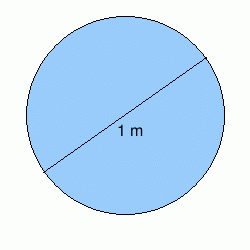Circumference: 2.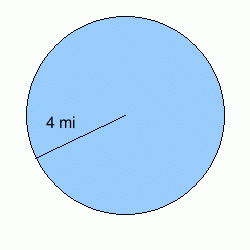Circumference:

### Complexity=6, Mode=exact

Find the circumference in terms of π and the unit of measurement. Type "pi" in for π so that "7π m" would look like "7pi m".

 1.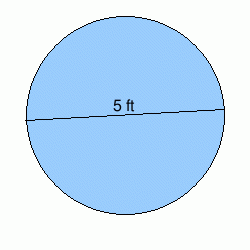Circumference: 2.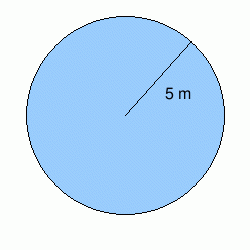Circumference:

### Complexity=8, Mode=exact

Find the circumference in terms of π and the unit of measurement. Type "pi" in for π so that "7π m" would look like "7pi m".

 1.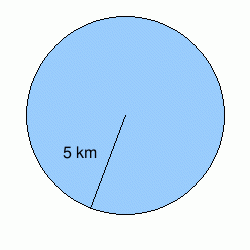Circumference: 2.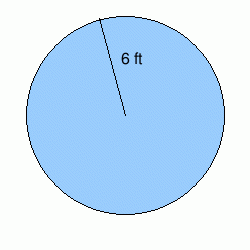Circumference:

### Complexity=4, Mode=fraction

Find the circumference. Use 22/7 as an approximation for π. A correct answer would look like 14.5 cm (use decimal if necessary).

 1.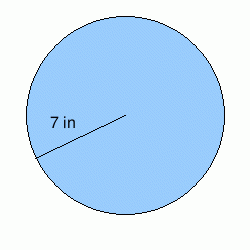Circumference: 2.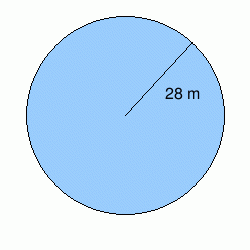Circumference:

### Complexity=4

Find the circumference. Use 3.14 as an approximation for π. A correct answer would look like 4.78 in.

 1.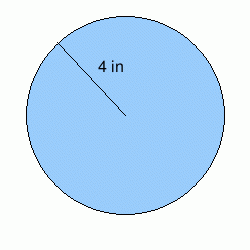Circumference: 2.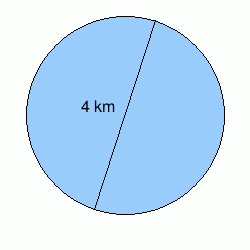Circumference:

### Complexity=4, Mode=exact

Find the circumference in terms of π and the unit of measurement. Type "pi" in for π so that "7π m" would look like "7pi m".

1Circumference:
Solution
Circumference = π × diameter
C = πd
Plugging values into the equation, we have:
C = π(1 m)
C = 1π m
2Circumference:
Solution
Circumference = π × diameter
C = πd
Since we have that the radius is 4 mi, we multiply it by 2 to get the diameter which then is 8 mi.
Plugging values into the equation, we have:
C = π(8 mi)
C = 8π mi

### Complexity=6, Mode=exact

Find the circumference in terms of π and the unit of measurement. Type "pi" in for π so that "7π m" would look like "7pi m".

1Circumference:
Solution
Circumference = π × diameter
C = πd
Plugging values into the equation, we have:
C = π(5 ft)
C = 5π ft
2Circumference:
Solution
Circumference = π × diameter
C = πd
Since we have that the radius is 5 m, we multiply it by 2 to get the diameter which then is 10 m.
Plugging values into the equation, we have:
C = π(10 m)
C = 10π m

### Complexity=8, Mode=exact

Find the circumference in terms of π and the unit of measurement. Type "pi" in for π so that "7π m" would look like "7pi m".

1Circumference:
Solution
Circumference = π × diameter
C = πd
Since we have that the radius is 5 km, we multiply it by 2 to get the diameter which then is 10 km.
Plugging values into the equation, we have:
C = π(10 km)
C = 10π km
2Circumference:
Solution
Circumference = π × diameter
C = πd
Since we have that the radius is 6 ft, we multiply it by 2 to get the diameter which then is 12 ft.
Plugging values into the equation, we have:
C = π(12 ft)
C = 12π ft

### Complexity=4, Mode=fraction

Find the circumference. Use 22/7 as an approximation for π. A correct answer would look like 14.5 cm (use decimal if necessary).

1Circumference:
Solution
Circumference = π × diameter
C = πd
Since we have that the radius is 7 in, we multiply it by 2 to get the diameter which then is 14 in.
Plugging values into the equation, we have:
C = π(14 in)
C = (22/7) × (14 in)
C = 44 in
Note that the 7 canceled out nicely for this specific radius.
2Circumference:
Solution
Circumference = π × diameter
C = πd
Since we have that the radius is 28 m, we multiply it by 2 to get the diameter which then is 56 m.
Plugging values into the equation, we have:
C = π(56 m)
C = (22/7) × (56 m)
C = 176 m
Note that the 7 canceled out nicely for this specific radius.

### Complexity=4

Find the circumference. Use 3.14 as an approximation for π. A correct answer would look like 4.78 in.

1Circumference:
Solution
Circumference = π × diameter
C = πd
Since we have that the radius is 4 in, we multiply it by 2 to get the diameter which then is 8 in.
Plugging values into the equation, we have:
C = π(8 in)
C = 3.14 × (8 in)
25.12 in
2Circumference:
Solution
Circumference = π × diameter
C = πd
Plugging values into the equation, we have:
C = π(4 km)
C = 3.14 × (4 km)
12.56 km